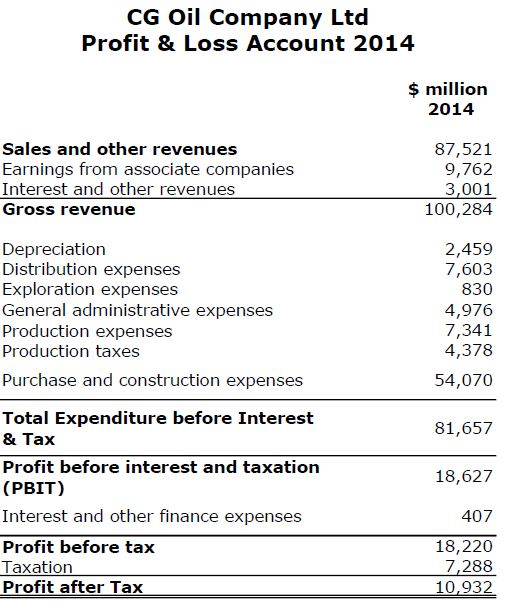# Key Financial Ratios Calculation Example

After learning about key financial ratio from the previous article (Key Financial Ratios To Evaluate Companies
), this article will show an example based on the financial statements of CG Oil Company in 2014.

Figure 1 and Figure 2 are a company balance sheet and profit and loss account, respectively.Figure 1 – CG Oil Company Balance SheetFigure 2 – CG Oil Company Profit and Loss

Company has a total of 10,000 million shares.

Company plans to pay a dividend of 0.4 \$/share.

These are calculations of the important financial ratios.

Current Ratio = Current Assets ÷ Current Liabilities

Current Ratio = 32,270 ÷ 36,843

Current Ratio = 0.876

This is not a good sign for this company since the current ratio is 0.875.

Quick Ratio = (Current Assets – Stock in Inventory) ÷ Current Liabilities

Quick Ratio = (32,270 – 8,745) ÷ 36,843

Quick Ratio = 0.639

Debt to Equity Ratio = Total Financial Debt ÷ Equity

Debt to Equity Ratio = (2,650 + 3,458) ÷ 29,892

Debt to Equity Ratio = 20.43%

Debt Ratio = Total Financial Debt ÷ Total Asset

Debt Ratio = (2,650 + 3,458) ÷ 79,653

Debt Ratio = 7.67 %

Profit Margin = Profit before interest and taxation (PBIT) ÷ Turnover (Sales)

Profit Margin = 18,627 ÷ 87,521

Profit Margin = 21.28%

You may need to compare it to other companies in the same business. This is a relative value. More profit margin = good.

Return on Asset (ROA) = Profit before interest and taxation (PBIT) ÷ Total Assets

Return on Asset (ROA) = 18,627 ÷ 79,653

Return on Asset (ROA) = 23.4%

Earnings Per Share (EPS) = Profit after Tax ÷ Total shares

Earnings Per Share (EPS) = 10,932÷ 10,000

Earnings Per Share (EPS) = 1.0932 \$/share

Dividend Cover = Earnings Per Share (EPS) ÷ Annual Dividend Per Share

Dividend Cover = 1.0932 ÷ 0.4

Dividend Cover = 2.733

Reference BookThomas Sowell (2007) Basic economics: A Common Sense Guide to the Economy, New York, New York, USA: Basic Books.

Share the joy
Tagged , . Bookmark the permalink.